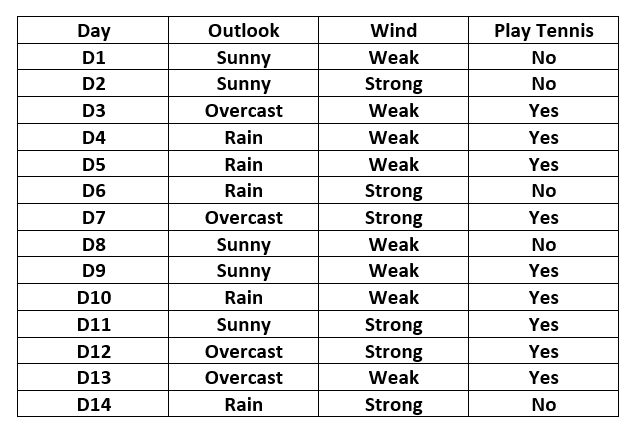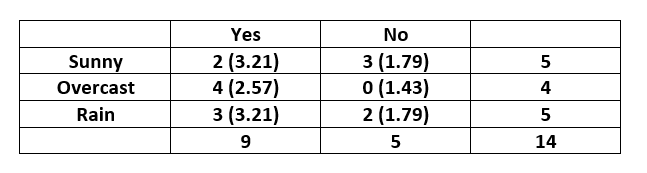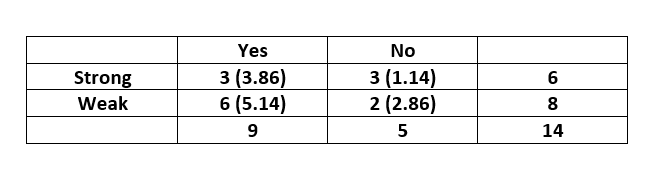GFG App
Open AppBrowser
Continue

# Chi-Square Test for Feature Selection – Mathematical Explanation

One of the primary tasks involved in any supervised Machine Learning venture is to select the best features from the given dataset to obtain the best results. One way to select these features is the Chi-Square Test. Mathematically, a Chi-Square test is done on two distributions two determine the level of similarity of their respective variances. In its null hypothesis, it assumes that the given distributions are independent. This test thus can be used to determine the best features for a given dataset by determining the features on which the output class label is most dependent. For each feature in the dataset, theis calculated and then ordered in descending order according to thevalue. The higher the value of, the more dependent the output label is on the feature and higher the importance the feature has on determining the output. Let the feature in question have m attribute values and the output have k class labels. Then the value ofis given by the following expression:

\chi ^{2} = \sum _{i=1}^{m} \sum _{j=1}^{k}\frac{(O_{ij}-E_{ij})^{2}}{E_{ij}}

where– Observed frequency– Expected frequency For each feature, a contingency table is created with m rows and k columns. Each cell (i,j) denotes the number of rows having attribute feature as i and class label as k. Thus each cell in this table denotes the observed frequency. To calculate the expected frequency for each cell, first, the proportion of the feature value in the total dataset is calculated and then it is multiplied by the total number of the current class label.

Solved Example: Consider the following table:Here the output variable is the column named “PlayTennis” which determines whether tennis was played on the given day given the weather conditions. The contingency table for the feature “Outlook” is constructed as below:-Note: Expected value for each cell is given inside the parenthesis. The expected value for the cell (Sunny,Yes) is calculated asand similarly for others. Thevalue is calculated as below:-[Tex]\Rightarrow \chi ^{2}_{outlook} = 3.129  [/Tex]

The contingency table for the feature “Wind” is constructed as below:Thevalue is calculated as below:-[Tex]\Rightarrow \chi ^{2}_{wind} = 3.629  [/Tex]On comparing the two scores, we can conclude that the feature “Wind” is more important to determine the output than the feature “Outlook”. This article demonstrates how to do feature selection using Chi-Square Test.

The chi-square test is a statistical method that can be used for feature selection in machine learning. It is used to determine whether there is a significant association between two categorical variables. In the context of feature selection, the chi-square test can be used to identify the features that are most strongly associated with the target variable.

Mathematically, the chi-square test involves calculating the chi-square statistic, which is a measure of the difference between the observed frequency of each category and the expected frequency under the null hypothesis of no association between the variables.

### The chi-square statistic is calculated as follows:

χ² = Σ((O - E)² / E)

where:

χ² is the chi-square statistic
O is the observed frequency of each category
E is the expected frequency of each category, which is calculated under the assumption of no association between the variables
The expected frequency for each category is calculated as follows:

E = (row total x column total) / grand total

where:

row total is the total number of observations in the row
column total is the total number of observations in the column
grand total is the total number of observations in the entire dataset
Once the chi-square statistic has been calculated for each feature, the p-value can be calculated using the chi-square distribution with (number of categories – 1) degrees of freedom. The p-value represents the probability of observing a chi-square statistic as extreme as the one calculated, assuming that there is no association between the variables.

Features with low p-values are considered to be more strongly associated with the target variable and are selected for further analysis or modeling.

In summary, the chi-square test is a statistical method that can be used for feature selection by measuring the association between categorical variables. The test involves calculating the chi-square statistic and p-value and selecting features with low p-values as being more strongly associated with the target variable.

### Advantages of using the chi-square test for feature selection include:

1. Simple and easy to use: The chi-square test is a simple and widely-used statistical method that can be easily applied for feature selection in machine learning.
2. Computationally efficient: The chi-square test is computationally efficient and can be applied to large datasets with many features.

My Personal Notes arrow_drop_up